Question
A ball is dropped from a height of 5 m. Can a motion map, v-t, or a-t graph be included as well please?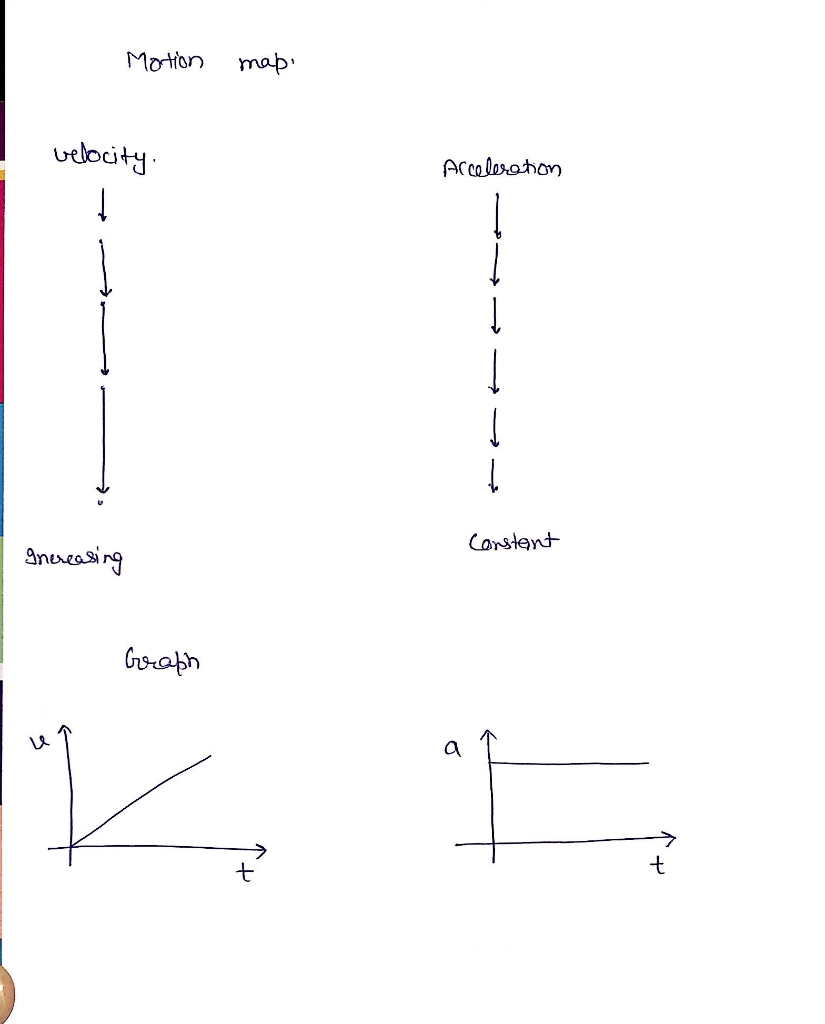#### Earn Coins

Coins can be redeemed for fabulous gifts.

Similar Homework Help Questions
• ### A tennis ball is dropped from a height of 5 m to the ground and the ball rebounded to a height of 4 m

A tennis ball is dropped from a height of 5 m to the ground and the ball rebounded to a height of 4 m. If the mass of the basketball is 1.0 kg and it is in contact with the floor for 50 ms, determine the average force exerted by the ground to the basketball.

• ### A red ball is dropped from rest at a height of 7.20 m. A blue ball...

A red ball is dropped from rest at a height of 7.20 m. A blue ball at a height of 12.0 m is thrown down at the same instant at 7.00 m/s. How long does it take the blue ball to catch up with the red ball? Please show the formula used and show every step that way I can practice a bunch of other problems like this one. Thank you so much! <3

• ### A 300 g ball is dropped from a height of 2.2 m , bounces on a...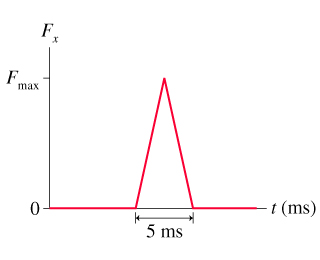A 300 g ball is dropped from a height of 2.2 m , bounces on a hard floor, and rebounds to a height of 1.7 m . The figure shows the impulse received from the floor. (Figure 1)What maximum force does the floor exert on the ball? Express your answer using two significant figures. max. 5 ms t (ms)

• ### A ball is dropped from a height 24 m above the ground at t=0.0. Each time...

A ball is dropped from a height 24 m above the ground at t=0.0. Each time it bounces from the ground, its rebound speed is 60% of its impact speed. At the instant the first ball hits the ground, a second ball is released from the same place. Take g = 9.8 m/s2. At what height above the ground will they collide? (Take the ground as y=0.0 m)

• ### A ball is dropped from a height 24 m above the ground at t=0.0. Each time...

A ball is dropped from a height 24 m above the ground at t=0.0. Each time it bounces from the ground, its rebound speed is 60% of its impact speed. At the instant the first ball hits the ground, a second ball is released from the same place. Take g = 9.8 m/s2. At what height above the ground will they collide? (Take the ground as y=0.0 m)

• ### A ball dropped from a height of 4.00-m makes a perfectly elastic collision with the ground....

A ball is dropped from a height of 4.00-m makes a perfectly elastic collision with the ground. Assuming no energy loss due to air resistance, (a) show that the motionis periodic and (b) determine the period of the motion. (c) Is the motion simple harmonic? Explain.

• ### A ball is dropped from rest at a height h. Directly below on the ground, a...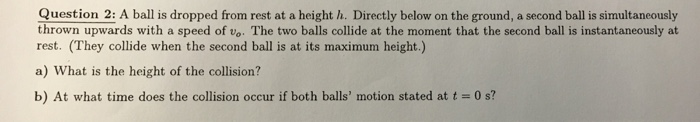A ball is dropped from rest at a height h. Directly below on the ground, a second ball is simultaneously t thrown upwards with a speed of vc. The two balls collide at the moment that the second ball is instantaneously at rest. (They collide when the second ball is at its maximum height.) What is the height of the collision? At what time does the collision occur if both balls' motion stated at t = 0 s?

• ### A ball dropped from a height of 4.10 m makes a perfectly elastic collision with the...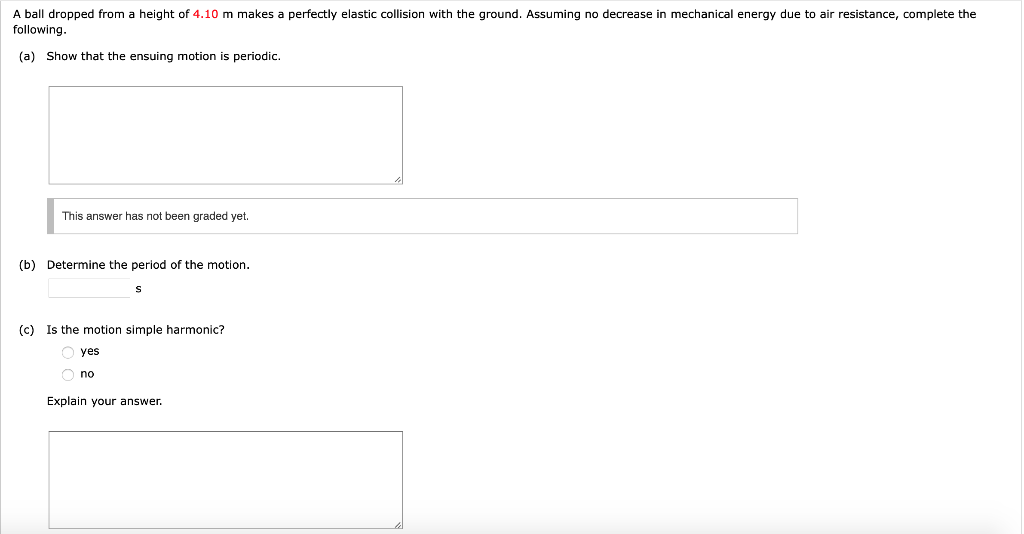A ball dropped from a height of 4.10 m makes a perfectly elastic collision with the ground. Assuming no decrease in mechanical energy due to air resistance, complete the following. (a) Show that the ensuing motion is periodic. This answer has not been graded yet. (b) Determine the period of the motion. (c) is the motion simple harmonic? yes no Explain your answer.

• ### (II) A ball is dropped from a height of 1.50 m and rebounds to a height of 1.20 m

Chap. 7, #36. (II) A ball is dropped from a height of 1.50 m and rebounds to a height of 1.20 m. Approximately how many rebounds will the ball make before losing 90% of its energy?

• ### INCREASING TIME > 6) Consider a ball that is dropped from rest from a height of...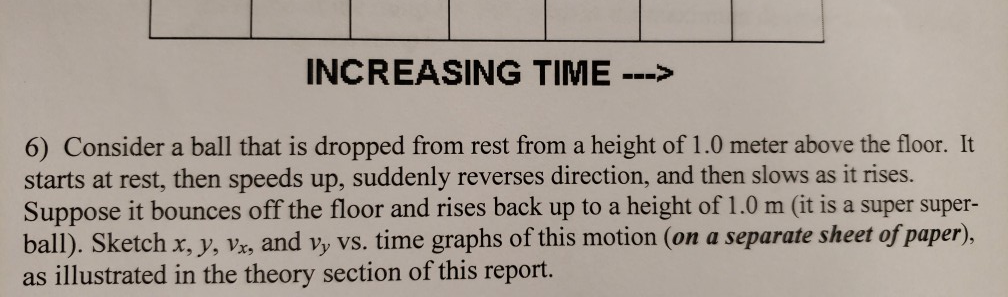INCREASING TIME > 6) Consider a ball that is dropped from rest from a height of 1.0 meter above the floor. It starts at rest, then speeds up, suddenly reverses direction, and then slows as it rises. Suppose it bounces off the floor and rises back up to a height of 1.0 m (it is a super super- ball). Sketch x, y, vr, and Vy vs. time graphs of this motion (on a separate sheet ofpaper), as illustrated in the...